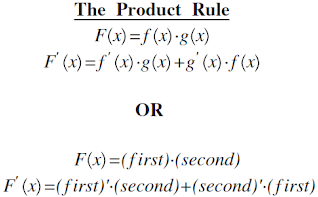# Differentiation

450 0

This is my first unit doing differentiation in Maths, and I feel so excited to step into the field of calculus. The concepts in this unit are indeed very different from before, but I enjoy the process of getting more and more used to it over time :) The following are the ways that I usually messed up on a question:

### Questions that I usually do wrong

Factorization

When using the product rule,After writing down the formula and when I am using the values of "first" and "second" to substitute the ones in the formula, factorization should always be done. That is when the problems occur. For example, when I am factorizing x3+ x, I tend to write x (x2+ 1) or something like that, just factorizing the thing wrong.

The Product Rule and the rule of differentiating power functions

Notice that the rule of differentiating power functions is:

If y = xn , then dy/d = nxn-1

When it comes to differentiating (3x+1)2 for example, I always take the final result as 2x, which is wrong. The product rule should be used and the final result should be 2x * 3 which equals 6x.